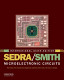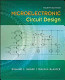### Electronics 1R

#### Course Description

Introduction. Basic characteristics of analog and digital circuits. Electrical characteristics of semiconductors, charge carriers, current conduction in semiconductors. pn and Schottky diode. Basic diode circuits in analog electronics (rectifiers, regulators, limiting circuits). MOS structure and MOSFET. Various FET types. Basic FET amplifier circuits. Basic CMOS logic circuits (inverter, NAND and NOR circuits, bistable multivibrator). Bipolar junction transistor (BJT). Basic BJT amplifier circuits. Differential amplifier. BJT switch. ECL logic circuits. Characteristics of ideal and real operational amplifier. Basic operational amplifier circuits in analog and digital electronics (amplifiers, multivibrators, triangular waveform generator). Analysis of electronic circuits by CAD tools.

#### General Competencies

Understanding of characteristics of electronic devices and basic circuits in analog and digital electronics. Understanding and usage of electronic circuits analysis techniques.

#### Learning Outcomes

1. explain the operation of basic semiconductor devices
2. analyze basic electronic amplifiers
3. compare the amplifiers with different electronic devices
4. analyze CMOS logic circuits
5. apply operational amplifier in analog and digital circuits
6. analyze of electronic circuits using electronic computers
7. apply basic measurements in electronic laboratory

#### Forms of Teaching

Lectures

lectures

Exercises

problem solving exercises

Laboratory Work

laboratory exercises

Consultations

consultations

Continuous Assessment Exam
Laboratory Exercises 0 % 12 % 0 % 12 %
Mid Term Exam: Written 0 % 44 % 0 %
Final Exam: Written 0 % 44 %
Exam: Written 0 % 50 %
Exam: Oral 38 %
##### Comment:

Before final exam students must have completed all laboratory exercises.

#### Week by Week Schedule

1. Role of electronics. Types of signals in analog and digital electronics. Impulse response of CR i RC networks
2. Electrical properties of semiconductors. Silicon structure. Intrinsic and doped silicon. Other semiconductors. Bandgap. Intrinsic concentration. Carrier concentrations. Temperature dependence. Carrier energy distribution.
3. Drift current. Semiconductor conductivity. pn-junction in equilibrium. Built-in voltage. Depletion layer.
4. Forward and reverse biased pn-junction. Current-voltage characteristics. pn-junction breakdown. Temperature dependence.
5. Small-sygnal diode resistance. Junction capacitance. Diffusion capacitance. Small-signal equivalent model. Diode as a switch. Metal-semiconductor junction. Schottky diode.
6. Diodes in optoelectronics: light emitting diodes, photodiodes, solar cells. MOS structure. MOS transistor. Operation. Current-voltage characteristics. CMOS structure.
7. FET temperature dependence and breakdowns. Small-signal FET parameters and small-signal equivalent model. FET amplifiers. Biasing.
8. Midterm exam.
9. Common-source, common gate and common drain amplifier. Comparison of basic FET amplifiers.
10. CMOS inverter: voltage transfer characteristic, time-domain operation, power dissipation. CMOS logic-gates. CMOS latches.
11. Bipolar junction transistor (BJT). Operation in the active mode. Current gains. BJT connections and modes of operation. BJT current-voltage characteristics. Temperature dependence.
12. BJT small-signal parameters and small-signal equivalent model. BJT amplifiers. Biasing. Common-emitter amplifier.
13. Common base and common collector amplifier. Comparison of basic BJT amplifiers.
14. BJT as a switch. ECL logic circuit. Ideal operational amplifier (Op-Amp). Properties of real operational amplifiers. Op-Amp amplifiers. Op-Amps in analog computations.
15. Final exam

#### Study Programmes

Computing (study)
Elective course from a group of mandatory courses-Electronics 1 (3. semester)

#### Literature

Ž. Butković, J. Divković-Pukšec, A. Barić (2013.), Elektronika 1, Fakutet elektrotehnike i računarstva, Zagreb - interna skriptaA.S. Sedra, K.C. Smith (2011.), Microelectronic Circuits, 6th ed., Oxford University PressR.C. Jaeger, T.N. Blalock (2011.), Microelectronic Circuit Design, 4th ed., McGraw-Hill

#### General

ID 141451
Winter semester
6 ECTS
L2 English Level
L1 e-Learning